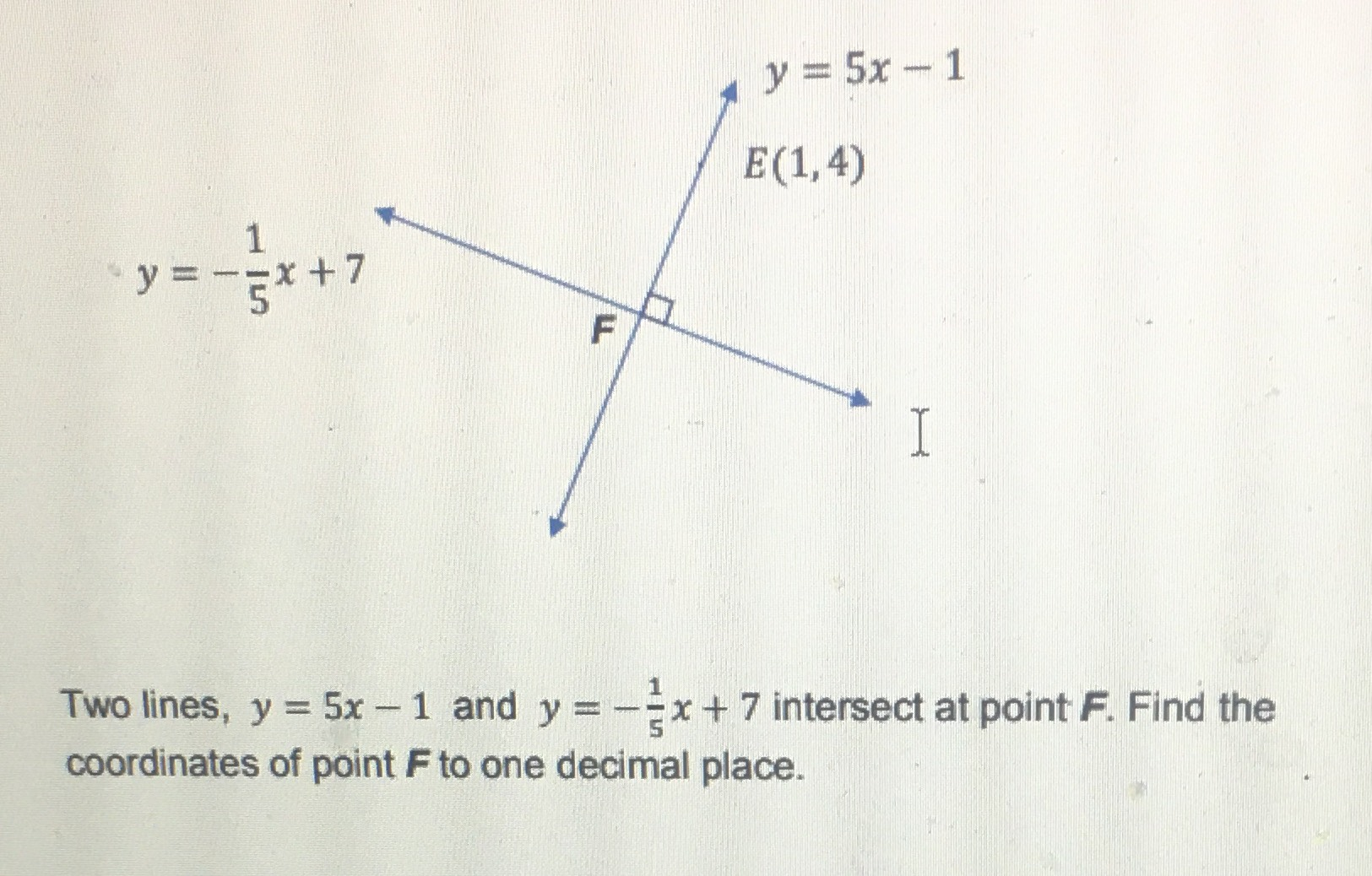### ¿Todavía tienes preguntas de matemáticas?

Pregunte a nuestros tutores expertos
Algebra
PreguntaTwo lines, $$y = 5 x - 1$$ and $$y = - \frac { 1 } { 5 } x + 7$$ intersect at point $$F$$ . Find the coordinates of point $$F$$ to one decimal place.

$$F(1.5,6.7)$$